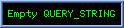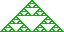Multivector Potential Theory

0-potentials
Classical and quantum scalar potentials are most naturally viewable as the e4 coordinate of 1-potentials of a slow Â3,1 flow. The classical 3D 1-vector potential providing the remaining coordinates. Central d-static potential ap = f(r)d where r=|p| and d2=±1 satisfies
Ñp2ap = ± (f"(r)+(N-1)r-1f'(r)) d according as p2 is ± .
f(r) = rg has f"(r)+(N-1)r-1f = rg-2(g(g-1)+(N-1)) vanishing when g(g-1) = N-1 ie. when g = 1 ± (4N-5)½ .

Newton Potential
To understand relativistic gravitation, it is helpful to understand the model of which it is essentially a refinement, Newtonian gravitation and, in particular, gravitational potential theory. In the Newtonian model, we can represent gravity by a 1-vector "acceleration" field GN(p) [  where p is a 4D Euclidean spacetime coordinate ] representating the instantaneous acceleration exerted on a small test mass placed at event p. Time is global in Newtonian physics so for a given t, we can regard G as a function of 3D spacial position r and GN is assumed to be irrotational (ie. (ÑrÙGN(r)) = 0) and so expressable as the gradient of a scalar field -f(r) known as the gravitational 0-potential. The minus sign ensures that the force ¦(r) = -Ñrf(r) tends to move matter from high to lower potential areas .
We can add an arbitary point-independant scalar constant to such a field without effecting its gradient and we typically seek to choose this constant so that f(r) ® 0 as |r|®¥ (in any direction).
A single point mass m at the origin generating an attractive gravitational field GN(r) = - gmr~(r-2) can be regarded as generating a 0-potential f(r) = -gm(r2) where point-independant scalar g is the universal gravitational constant. Let V be an arbitary volume (3-curve) having boundary surface (2-curve) dV. By Gauss's law, the integral of GN(r) over dV is zero unless 0 Î V in which case it is -4pgm (regardless of the geommetry of V) . In general, if scalar mr is the local "mass density" at r, assumed to be a continuous function of r, we have
òdV GN(r)¿dr2 = -4pgòV mr dr3
Now òdV GN(r)¿dr2 = òV (Ñ¿GN(r))|dr3| by the divergence theorem so for arbitary small V we have
òV (Ñ¿GN(r)-4pmr)|dr3| = 0 whence Ñ¿GN(r) = -4pgmr and so
Ñr2f(r) = Ñr¿(Ñrf(r)) = -4pgmr       which (for scalar f(r)) is known as Poisson's equation , or Laplace's equation if mr=0 .

If f(r) = f(|r|) = f(r) we have Ñrf(r) = f'(r)r-1r = f'(r)r~ and Ñr2f(r) = r-1f'(r) + f"(r).

This then is the Newtonian model. The 3D gravitational force field G(r) is characterised by it's scalar "integral" f(r) = -Ñr-1 G(r) ; a 0-potential scalar field which satisfies Poissons equation everywhere and provides the acceleration on a test mass at r as GN(r)=-Ñrf(r) . We can in principle compute f(r0) as the infinite volume integral
f(r0) = gòÂ3 mr(r-r0) |dr3|
The scalar field mr fully determines GN so the "flow" of matter is relevant in determining the instantanous gravitational potential only by its magnitude. It's direction effects only how GN changes over time.

Gravity is such that the force exerted on a test particle is proportional to the mass of the test particle so that the acceleration experieneced is independent of the mass, More generally we have a force field Fp = Ñpfp .

Effective Potential
Under a central force with classical 0-potential f(r)=f(r) , the 2-blade angular momentum L=mrÙr' and scalar energy Em(r')2 + V(r) are both conserved and the radial energy equation ½m(r')2 + V(r) - ½m-1r-2L2 = E for r³0 implies that the shape of the 1D scalar effective potential f(r)   - ½m-1r-2L2 = f(r) + ½m-1r-2L2 determine the possible orbits for a given L=|L2|½ and E combination. If the particle is released from rest L=0 , but otherwise the effective potential contains a term equivalent to that contributed by a repulsive inverse cube force m-1L2 r-3

Orbital Stability Indicator

Suppose a particle of inertia m is subject to a time t-independant central force ¦(r,t)r~ =  ¦(r)r~.
If x=r-r0 denotes the deviation of r from cirular orbit value r0 then mx.. » (r0-13¦(r0)+¦'(r0))x to first order in r-1x where .. denotes second time derivative. For gravitationl forces ¦(r) is proportionate to the inertia m.

mr.. = ¦(r)+L2r-3 where angular momentum L=mr2q. is conserved.
Let x=r-r0. We have mx.. = ¦(r0+x) + L2(r0+x)-3 » ¦(r0)+x¦'(r0)+½x2¦"(r0) + 3!-1¦"'(r0)x3 + ... + r0-3L2(1-3r0-1x + 6r0-2x2 - 10r0-3x3 +...
For circular orbit at r0 we require L2 = -mr03¦(r0) , w = (-r0-1m-1¦(r0))½ so
mx.. » ¦(r0)+x¦'(r0)+½x2¦"(r0) + 3!-1¦"'(r0)x3 + ... - ¦(r0)(1-3r0-1x + 6r0-2x2 - 10r0-3x3 +...)
= x¦'(r0)+½x2¦"(r0) + 3!-1¦"'(r0)x3 + ... + ¦(r0)(3r0-1x - 6r0-2x2 + 10r0-3x3 +...)
= x(¦'(r0) + 3r0-1¦(r0)) + x2( ½¦"(r0) - 6r0-2) + x3(3!-1¦"'(r0) + 10r0-3) + O(x4)

Thus if orbital stability indicator OSI(r0) º -3r0-1¦(r0) - ¦'(r0)   =   3r0-1f'(r0) + f"(r0)  is positive we have oscillations about the circular orbit of frequency m OSI(r0)½ and so period 2pm½ OSI(r0) , a proportion (-3-r0¦'(r0)¦(r0)-1) of the orbit period 2p(-r0-1m-1¦(r0)), while if OSI(r0) is negative we have hyperbolic deviation.
We will see below that OSI(r) = 2r-1E'(r) where E(r)=f(r)+½rf'(r) is the total energy of a circular orbit of radius r.

If ¦'(r0) + 3r0-1¦(r0) vanishes, we have harmonic deviation equation mx" » x2( ½¦"(r0) - 6r0-2) = -PSI(r0)x2 where
PSI(r0) º r0-2(6+½r02f"'(r0)) = 6r0-2 + ½f"'(r0) .
This is analytically problematic since while x" = ax2 has a particular known solution x(t)=6a-1(t+6a-1x(0)-1)-2 this is not general enough to match a given x'(0) .

The normalised orbilital stability indicator of a circular orbit of radius r0 under a central force ¦(r) £ 0  is the value
OSI(r0) º (3+r0¦(r0)-1¦'(r0))½ , where ½ denotes that for negative values we take the negative square root of the absolute value. In eliminating the magnitude of ¦ by multiplying OSI(r) by |r-1¦(r0)|½, we aquire an infinite OSI(r) when ¦(r)=0. The sign of ¦(r) has also been eliminated so OSI(r) of a repuslive central force is the OSI of the attactive force -¦(r). For nonzero ¦(r), OSI(r) is the number of orbits about 0 per peturbation oscillation.
OSI(r) provides a measure of deviation from shell condition p(t)2 = p(0)2 under acceleration p"(t) = -f'(|p(t)|)p(t)~ where |p(t)| º |p(t)2|½, assuming p(0)¿p'(0) is small. Large OSI suggests rapid oscillations across the shell with integer values suggesting petal forms. OSI<1 suggesting slowly corrected slightly noncircular orbits.

"Power law" attractive force f(r)=-ark has OSI(r) = (3+k)½ so stable circular orbits require k>-3 . Inverse square forces have unit OSI(r), so the period of the deviation coincides with that of the orbit, stretching it into an ellipse for example. An integer OSI(r) indicate a single repeated orbit while rational OSI(r) pq-1 implies that after q orbits each of which contain p deviation cycles the trajectory will repeat itself. An r-1 orbit takes ½½ orbits to complete an oscillation and, as this is irrational, the trajectory in theory never repeats. However, as the oscillatory nature of deviations is only correct to first order in small deviations, such multi-orbit analysis is profoundly suspect.
High OSI(r) indicate rapid oscillations about the circular orbit while high negative OSI indicate rapid hyperbolic deviations, with the caviat that ¦(r) changing sign gives infinite OSI when ¦(r)=0 corresponding to deviations from the straight line zero forces trajectory. Zero OSI indicates paraboloid deviations from u with q correspending to paraboloid deviation from u0 with q while OSI j as for ¦(r)=-arj2-3 corresponds to oscillating j times faster than the orbit.

To maximise OSI(r) º r-13f'(r) + f"(r) we consider OSI'(r) =  -3r-2f'(r) + 3r-1f"(r) + f"'(r) which is zero when f"'(r) =  3r-2(f'(r) - rf"(r)) whereupon PSI(r) = r-2(6+(3/2)(f'(r) - rf"(r))) = (3/2)r-2(4 + f'(r) - rf"(r)) .

PSI'(r) = -12r-3 + ½f(4)(r) vanishes everywhere for f(r) = 6r(ar) + P4(r) = 6(ar + 6r(r) + P4(r) for arbitary a and quartic polynomial P4() with
f'(r) = 6(ar) + 6 + P4'(r).
f"(r) = 6r-1 + P4"(r).
f"'(r) = -6r-2 + P4"'(r).
f"(r)+(N-1)r-1f'(r)-(N-1)

OSI(r0)= 3r0-1f'(r0) + f"(r0) = 18r0-1(ar0) + 18_roinv + 3P4"(r) + 6r-1 + P4"(r). = 18r0-1(ar0) + 24_roinv + 4P4"(r) .
ò0r0 dr 6r(ar) = 6 ò0ar0 dx a-1x (x) = 6a-1 [ x2(½(x)-¼) ]0ar0   =   6ar02(½(ar0)-¼) .

However, this is less intersting than PSI'(r)=0 coinciding with OSI'(r)=0 and PSI(r)=0 .

Stability Indicators of a Klein Gordan 0-Potential

If Ñx2f(r) = af(r) then f"(r) = ±af(r) - (N-1)r-1f'(r) according as x2 is ±, and so   OSI(r) = (4-N)r-1f'(r) ± af(r) and for N=4 we have OSI(r) = ±af(r) .
OSI'(r) = (±a - (4-N)r-2)f'(r) + (4-N)r-1 f"(r)
= (±a - (4-N)r-2)f'(r) + (4-N)r-1 (±af(r) - (N-1)r-1f'(r))
= (±a - (4-N)r-2 - (4-N)(N-1)r-2)f'(r) ±a(4-N)r-1f(r)
= (±a - N(4-N)r-2)f'(r) ±a(4-N)r-1f(r) .
Thus OSI(-r) is extreme when
= f'(r) = -/+a(4-N)r-1(±a - N(4-N)r-2)-1 f(r) .
Further, f"'(r) = ±af'(r) - (N-1)r-1 (±af(r) - (N-1)r-1f'(r)) + (N-1)r-2f'(r)   =   (±a + N(N-1)r-2)f'(r) -/+a(N-1)r-1f(r) according as x2 is ±; and so
PSI(r) = r-2(6 + ½r2f"'(r)) =
OSI'(r) =  -3r-2f'(r) + 3r-1f"(r) + f"'(r)   =    -3r-2f'(r) + 3r-1( ±af(r)-(N-1)r-1f'(r) ) + (±a + N(N-1)r-2)f'(r) -/+a(N-1)r-1f(r)

Circular Orbits

-f'(r)r~ = -mw2r for inertial mass m>0 yields w = ±|m-1r-1f'(r)|½ Þ wr = ±m |rf'(r)|½ , and so circular orbits under central force ¦(r)r~ = -f'(r)r~ have

• period 2pw-1 = 2p|m-1r-1f'(r)| = 2pm½|rf'(r)-1|½   ;
• speed rw = ±|m-1rf'(r)|½     and so sublight orbits require |rf'(r)| < m ;
• classical kinetic energy ½mw2r2 = ½rf'(r) ;
• total energy E(r) = f(r) + ½rf'(r) with E'(r) = ½(3f'(r) + rf"(r)) = ½rOSI(r) ;
• angular momentum mr2w = m½r3/2f'(r)½ = |mr3f'(r)|½ ;
• radial acceleration -rw2 = m-1f'(r)

The potential f(r)=-lrb with l>0 has f'(r)=br-1f(r) and hence squared angular momentum mlbrb+2 and kinetic energy |bf(r)|. For b<0 the toal orbit energy is thus (b+1)f(r) and so in a Coloumb potential with b=-1 all circular orbits have total energy zero, while if b=-2 all circular orbits have angular momentum (2ml)_sqdrtcnj and positve total energy -f(r).

The Coulomb Potential f(r)=-lr-1 with f'(r)=lr-2 thus has w = ±|m-1lr-3|½ ; period 2p|m½l r3/2 ; speed  ±|m-1lr-1|½ ; angular momentum ± m½r½ ; classical kinetic energy ½lr-1 ; and radial acceleration -m-1lr-2.
OSI(r) = r-13f'(r) + f"(r) = -lr-3 while OSI(r) = 1.

More generally a small test particle launched from r0e1 with velocity r0w0e2 + s0e1 will seek a circular orbit having mr02w0 = mr3/2f'(r)½ and f(r0) + ½m(r02w02+s02) = f(r) + ½rf'(r) .

Quantised Orbits
There are two natural ways to particularise orbits under a central oscillatory potential f(r). The maxima of OSI(r) provide the stablest orbits, while orbits at r satisfying |rf'(r)| = mv2 provide the orbits having a given speed v. In particular, v=1 yeilds the lightspeed orbits with inertial mass m regarded as a coulping constant rather than a mass-energy.

Ring Potential
The ring potential foRz(r) º 2R ò0p dq f((z2+r2+R2-2rR cosq)½) is the potential at r of a circle of radius R lieing in a plane containing 0 and r.
Setting s º  (z2+r2+R2-2rR cos(q)) we have ds = 2rR sinq dq.
cos(q) = (2rR)-1(s-z2-r2-R2) so for q Î [0,p] we have
sin(q) = (2rR)-1 (4r2R2 - (s - z2-r2-R2)2)½ = (2rR)-1 (2s(z2+r2+R2) -s2 + 4r2R2 - (z2+r2+R2)2)½    = (2rR)-1 Q(s)½ where
Q(s) º -(s2 - 2s(z2+r2+R2)   + (z2+ r2+R2)2   - 4r2R2 )
= -s2 + 2s(z2+r2+R2) - z4 - 2z2(r2+R2) - (r2-R2)2
=?= -(s-z2-(r-R)2)(s-z2-(r+R)2) is a quadratic in s with roots z2 + (r±R)2.
Thus foR(r) = 2r-1 òz2+(r-R)2z2+(r+R)2 ds f(s½) ( sinq)-1 = 4R ò(r-R)2 (r+R)2 ds Q(s) f(s½) .

For z=0 , Q(s) = -(s2 - 2s| (r2+R2) + (r2-R2)2 ) ,

Morse Potential
Typically used for r representing the seperation between two covalently bonded atoms, f(r) = f¥(1-(-(½kf¥-1)½(r-r0)))2     for dissassociation energy f¥>0, equilibrium or zero force distance r0, and bond force constant k>0.
 Yellow shows potential f(r); green is force f'(r); while red is the OSI (3+f'(r)-1rf"(r))½ with imiginary values focred negative real.     For rÎ[0,r0] we have f(r)£f0=f(0)= f¥(1-((½kf¥-1)½r0)↑)2 with f(r)³f¥ for r£ r0 - (2f¥k-1)½(2)↓.     f(r) ® f¥ from below as r®¥ and to ¥ as r®-¥ though we might typically impose r³0. We have a global minimum at r=r0 with f(r0)=0 , f'(r0)=0, and f"(r0)=k.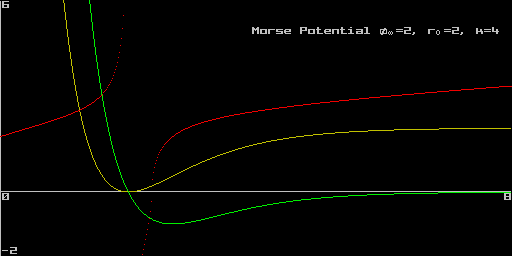[ Proof :  Set m(r) = (-(½kf¥-1)½(r-r0)) = 1-f¥f(r)½ so that m(r0)=1 and f(r)=f¥(1-m(r))2 with m(k)(r) = (-1)kkf¥-1)½km(r)
f'(r) = -2f¥(1-m(r))m'(r) = (2k)½f(r)½ m(r) Þ f"(r) = 2f¥(m'(r)2 -(1-m(r))m"(r))   = km(r)(2m(r)-1)  .]
Ñ2f(r)=r-1f'(r)+f"(r) = r-1(2k)½f(r)½ m(r) + km(r)(2m(r)-1) = m(r)(r-1(2k)½f(r)½ + k(2m(r)-1))

The comnplex Morse potential f(r) = b2(-2(r-r0)) - b(1+2D)(-(r-r0)) = (b(-(r-r0)) - (½+D))2 - (½+D)2 gives real energy eigenvalues En=-(n-D)2 for integer n<D. [Saaidi]

Born Mayer Potential
The Born-Mayer potential is the exponentiion f(r) = f0 (-ar) with with a>0. Since f(k) = (-a)k f(r) we have OSI(r) = a(a+3r-1) f(r) ; OSI(r) = (3+ar)½ ; and PSI(r) = 6r-2 - ½a3 f0(-ar) zero when r-2(ar) = 12-1a3f0-1 .

Lennard-Jones Potential
The Lennard-Jones potential is a convenient fiction used to approximate potentials that strongly repel close up but attract at a distance. f(r) = 2fr0(r0-1r)-6(1 - ½(r-1r)-6) where fr0<0 .
This is +¥ at r=0, 0 at at 26-1r0 » 1.12246r0 and ®0 as r®¥. Since f'(r) = 12fr0r-1(r0-1r)-6(1 - (r-1r)-6) , f(r) is minimised at r=r0 by f(r0) = fr0.

Tangential occlusion potential
Constructing an inverse square particle-mediated field is unrealistic because the r-1 factor representing an inverse square "dispersion" of a constant signal fails for low r . If the absorbing particle is not attracting the carriers but merely absorbing those that reach a small perimeter distance h , then we would expect the proportion of carriers absorbed to be in proportion to the area content of the spherical cap defined by the cone tangential to the h radius sphere centered on the absorber. A sphere of radius h at distance r from 0 subtends a cone of demiangle q= sin-1(r-1h) = sin-1(u) where uºhr-1 , which occludes an area 2p(1- cos(q))d2 = 2p(1-(1-u2)½)d2 of the 4pd2 surface area of a sphere of radius d at 0, rather than an area proportionate to r-2.
 Attractive central force     ¦(r) = (1-(hr-1)2)½ - 1 = (1-u2)½ - 1 for r³h (ie. u £1) has     ¦'(r) = h2r-3(1-u2)-½ = h-1u3(1-u2)-½ = h2r-3(1+¦(r))-1 .     For r>>h we have ¦(r) » -½(hr-1)2 - 1/8(hr-1)4   - K3(hr-1)6 - K4(hr-1)8 - ...   where Kk º 1*3*5*7*...*(_2k-3) / (2*4*6*8*...*(2k)) = 22-2k(2k-3)! / ((k-2)! k! ) .      For large r (so small u=hr-1), the inverse square "Coulomb" force will be massively dominant.     We find that circular orbits are stable only for   u2< 3/4 (ie. r>(3-½)2h) and that the 3 + r¦'(r)¦(r)-1 indicater tends to 1 from below as r®¥ .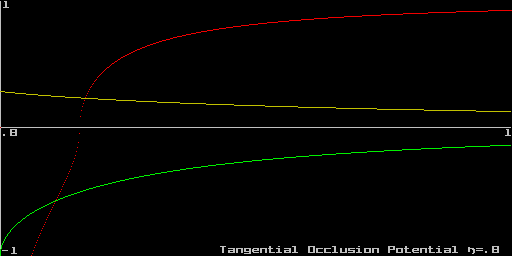For a screened force F(r) = (-lr) ¦(r) we have Z(F)(r) = F(r) + 3-1rF'(r)   =   (-lr) ( Z(¦)(r) - 3-1lr¦(r) ) . Now -3-1lr¦(r) is positive so exponentially damping ¦ makes circular orbits unstable whenever
-1 + (1-u2)(1-(2/3)u2) - 3-1lr((1-u2)½-1) ³ 0 Û -1 + (1-u2)(1-(2/3)u2) + 3-1lr ³ 3-1lr(1-u2)½ Û -1 + 3-1lr ³ (3-1lr - 1 + (2/3)u2)(1-u2)½

Comparing this with indicater (1-3-1)rk for ¦(r)=rk we see that for large r, circular orbits are only just stable; verging on the the instability associated with inverse cube orbits.
The apsidal angle p(3+r¦'(r)¦(r)-1)   =   p(3 + d2r-2(1-u2) (-1 + (1-u2)½)-1 )   =   p(3 + u2( (1-u2)½ (-1 + (1-u2)½) )-1 )   =   p(3 + u2( 1-u2-(1-u2)½ )-1 )
When u2=½ this is p(3 + ½( ½ - (½)½ )-1 ) =p(3 + (1-2½)-1 ) » 1.30p .
It is a rational multiple of p only when 3 + u2( 1-u2-(1-u2)½ )-1 is a squared rational number, which occurs when  1-u2-(1-u2)½ is a squared rational, .

We will here refer to V(r) = òr¥ dr ¦(r)   =      r¦(r) + h sin-1(hr-1)   =      r((1-(hr-1)2)½-1) + h sin-1(hr-1)   =      h(u-1((1-u2)½-1) + sin-1(u)) as the tangential occlusion potential.
[ Proof :   òr¥ dr ¦(r)   =   òu0 (-r2h-1 du) ¦(r)   =   h òu0 du -u-2¦(r)   =      h ò0u du ((1-u2)½ - 1)u-2 =   h [ -(1-u2)½u-1 - sin-1(u) + u-1 ]0,u   =     h(u-1(1-(1-u2)½) - sin-1(u))   =      -r¦(r) - h sin-1(hr-1)  .]

(/r)2V + 2r-1V/r   =   -r-1(1-u2)¦(r)2
[ Proof :  V/r = -¦(r) and (/r)2V + 2r-1V/r   =     =   -¦'(r) + 2r-1¦(r)   =   -h2r-3(1-u2) + 2r-1((1-u2)½-1)   =   r-1(1-u2)(-u2 + 2(1-u2) - 2(1-u2)½)   =   r-1(1-u2)(2-u2 - 2(1-u2)½)   =   r-1(1-u2)(1 - (1-u2)½)2   =   -r-1(1-u2)¦(r)2  .]

Setting V(r) = (1-u2)a gives V/r = a(1-u2)(a-1)2h2r-3 so r2 V/r = 2h2a(1-u2)(a-1)r-1 whence
Ñ2 V(r) = r-2 (/r)(r2 V/r)   =   2r-2h2a ( (a-1)(1-u2)(a-2)2h2r-4 - (1-u2)(a-1)r-2)
=    2r-4h2a (1-u2)(a-2)( (a-1)2u2 - (1-u2) )   =    2r-4h2a (1-u2)(a-2)( (2a-1)u2  - 1 )

Yukawa potential
f(r)=rb(arg) has
f'(r) = (garg-1+br-1)f(r) ;
f"(r) = (g(g-1)arg-2-br-2)f(r) + (garg-1+br-1)f'(r)   =   r-2(g(g-1)arg-b + (garg+b)2 )f(r)   =   r-2(g2a2r2g + g(g-1+2b)arg + b(b-1))f(r)
Thus f"(r)+(N-1)r-1f'(r)   =   (g(g-1)arg-2-br-2)f(r) + ((garg-1+br-1 + (N-1)r-1)f'(r)
=   r-2(g(g-1)arg-b + (garg+b+N-1) (garg+b))f(r)
=   r-2(g(g-1)arg-b + g2a2r2g + garg(2b+g+N-1) + b(b+N-1)f(r)
=   r-2( g2a2r2g + garg(g+2b+N-2) + b(b+N-2))f(r)

Setting b=2-N, g=N-2 gives f"(r)+(N-1)r-1f'(r) = (N-2)2a2r2(N-3)f(r), thus for N=3 we have central f(r)=r-1(ar) solving Klien Gordan equation Ñp2f =a2f.
 Taking a=-m<0, b=-1, g=1, d=0 gives the   real scalar Yakuwa potential or screened-Coulomb potential ±(-mr)↑ r-1 with Ñx2 (-mr)↑ r-1 = m2 (-mr)↑ r-1 .     This can be loosely regarded as the potential for a putative inverse square force (with negative potential generating an attractive force) mediated by decaying carriers, although it actually only approximates òR¥  dr r-2(-mr)↑ = (-mS)↑R-1  + m-1E(-m-1R) where E(s) º ò-¥s dt t-1(t)↑ is the integral exponential function, small for large negative s. [ Proof :  Setting s=-mr: -m ò-mR-¥  ds s-2(s)↑ = m(- [(s)↑s-1]-¥-mR + ò-¥ -mS  ds s-1(s)↑ = (-mS)↑R-1  + mEi(-m-1R)  .]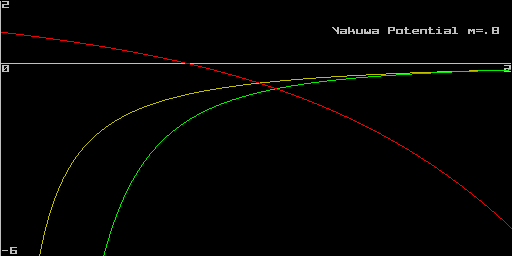To explain the strong nucleonic (proton and neutron) forces, observed to act at a range of about R=10-15 m, Yakuwa postulated massive mediator particles of lifetime Dt = c-1R. Uncertainty principle DEDt ³ h then implies DEc-1R ³ h with uncertainty minimised by DE = chR-1 » 200 MeV. Historically first the muon, then the pion, were regarded as filling this role, necessitating a high coupling constant of about 14, making peturbation theories neglecting higher order couplings inapplicable for the strong nucleonic forces.

Trigonometric Potential
Taking a = ia gives f(r)=r-1(ari) with Ñx2f = -a2f .
 f1(r) = r-1 sin(ar) = a Sin(Q)     f'(r) = -r-2 sin(ar) + ar-1 cos(ar) = ar-1( cos(Q)= Sin(Q))     f"(r) = (2r-3-a2r-1) sin(ar) - 2ar-2 cos(ar)     = ar-2(-2 cos(ar) + (2(ar)-1   - ar) sin(ar))     OSI(r) = ar-2( cos(ar) - R-a,N sin(ar))       =   ar-2 ((ar)2 + (ar)-2 + 3)½ sin(ar- cot-1(R-a,N)) where R-a,N=ar+(ar)-1.     For small ar, f1(r) = a - 3!-1a3r2 + O(r-1(ar)5)   is approximately harmonic.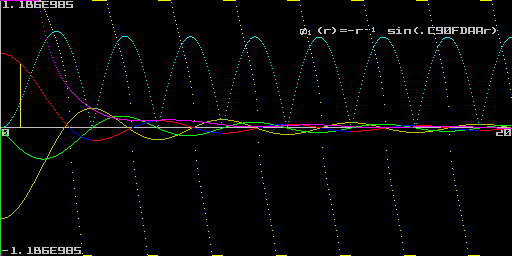OSI(r) is extremal when Q -   tan-1(3-1Q(3+Q2)) = kp which is a condition of Q independant of a. [ Proof : OSI'(r) = 2ar-3( - cos(ar) + (ar + (ar)-1) sin(ar) ) - ar-2( +a sin(ar) + (a - a-1r-2) sin(ar) + a(ar + (ar)-1) cos(ar) )     = ar-3( -2 cos(ar) + 2(ar + (ar)-1) sin(ar)   - ( +ar sin(ar) + (ar - (ar)-1) sin(ar) + ar(ar + (ar)-1) cos(ar) )     = ar-3( (-2-ar(ar+(ar)-1)) cos(ar) + (2(ar + (ar)-1) - ar - (ar - (ar)-1) ) sin(ar) )     = ar-3( -(3+(ar)2) cos(ar) + 3(ar)-1 sin(ar) )   =   a4Q-3( -(3+Q2) cos(Q) + 3Q-1 sin(Q) )     = a4Q-3 ( (3+Q2)2 + 9Q-2)½ sin(Q -   tan-1(3-1Q(3+Q2))  .]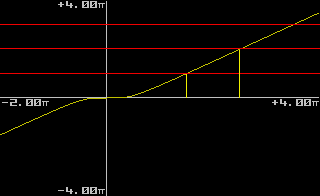Define Wk to solve x - tan-1(1/3Q(3+Q2)) = kp .     [spctime1]

E(r) = ½(a cos(ar)- Sin(ar))

Logarithmic Potential
f(r) = rg(arb) + m has f'(r) = rg-1(g(arb)+b) ; f"(r) = rg-2((g-1)g(arb)+(2g-1)b) so that f"(r)+(N-1)r-1f'(r). = rg-2((g+N-2)g(arb)+(2g+N-2)b)
Setting g=2 gives f(r) = r2(arb) + ½N-1(N+2)b = r2(a + br) + ½N-1(N+2)b with f'(r) = = r(2(arb)+b) solving Ñx2 f(r) = ±2Nf(r) for any a,b according as x2 is ±.
OSI(r) = (4-N)r-1f'(r) ± 2Nf(r) = (4-N)(2(arb)+b) ± (2Nr2(arb) + N(N+2)b) = 2((4-N) ± Nr2)(arb).

Next : 1-Potentials

Glossary   Contents   Author
Copyright (c) Ian C G Bell 1999
Web Source: www.iancgbell.clara.net/maths
Latest Edit: 14 Jun 2014.